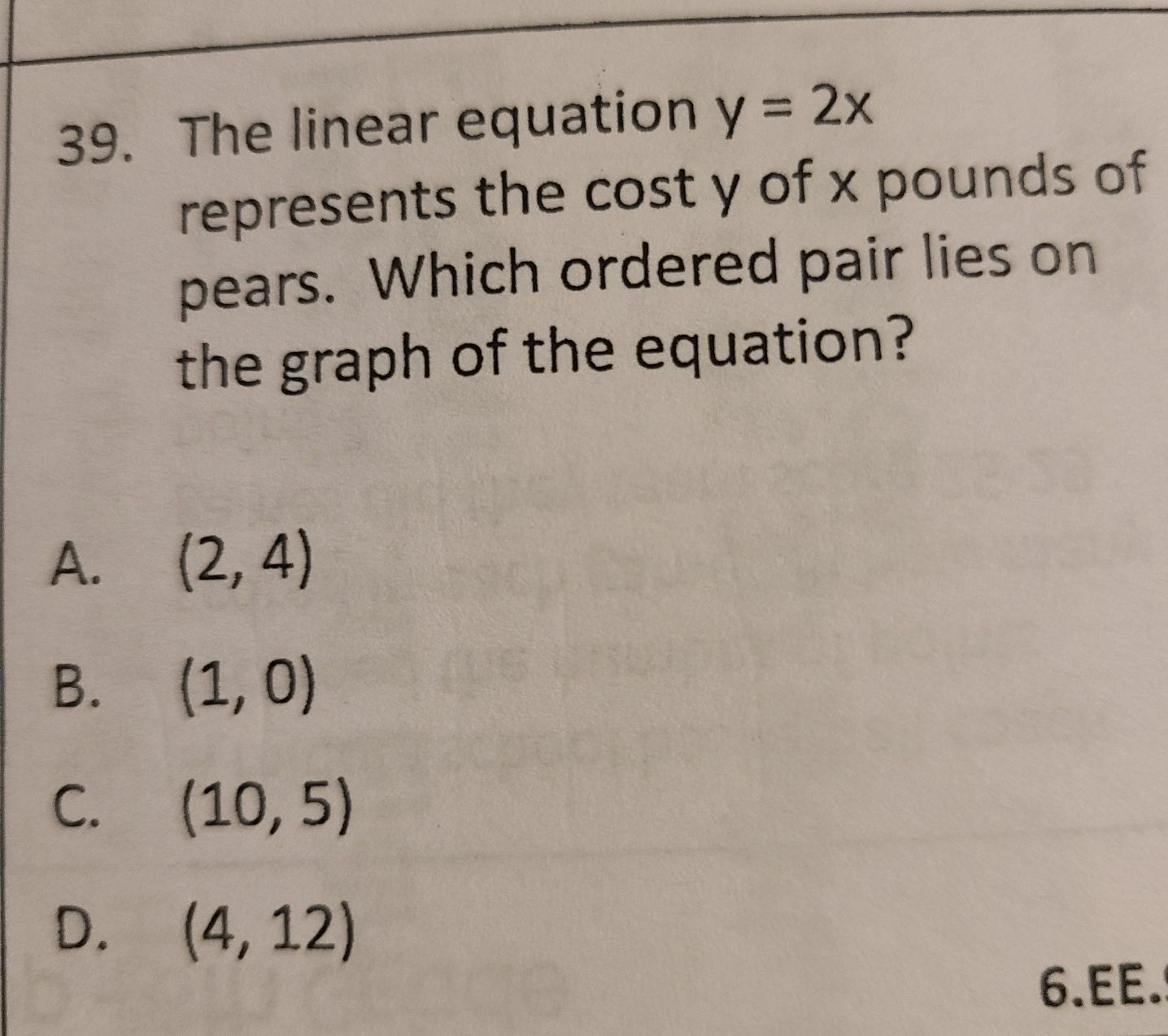### ¿Todavía tienes preguntas de matemáticas?

Pregunte a nuestros tutores expertos
Algebra
Pregunta39. The linear equation $$y = 2 x$$ represents the cost $$y$$ of $$x$$ pounds of pears. Which ordered pair lies on the graph of the equation?\

A. $$( 2,4 )$$

B. $$( 1,0 )$$

C. $$( 10,5 )$$

D. $$( 4,12 )$$